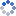加载中…夜晚的虫子
• 博客等级：• 博客积分：0
• 博客访问：18,484
• 关注人气：3
• 获赠金笔：0支
• 赠出金笔：0支
• 荣誉徽章：

## 最小割 Stoer-Wagner 算法(2011-06-09 20:31:37)

### 杂谈

G的任意s-t最小割Min-Cst）：

st是途中的两个点且边（st）∈E（即st之间存在一条边）。如果G的最小割CutG分成MN两个点集

①：如果sMtNMin-Cst= Cut（不讨论）

②：如果stM（或者stN）则Min-Cst<= Cut

s-t最小割的方法

1.令集合A={a}aV中任意点

2.选取V-A中的w(A,x)最大的点x加入集合

3.|A|=|V|，结束，否则更新w(A,x)，转到2

Poj (pku) 2914 Minimum CutGVE

V-A直到为空

w[]的情况如下

 w[i] 0 1 2 3 4 5 6 7 初始值 0 0 0 0 0 0 0 0 A=A∪{0} --- 1 1 1 1 0 0 0 A=A∪{1} --- 2 2 1 0 0 0 A=A∪{2} --- 3 1 0 0 0 A=A∪{3} --- 1 0 0 1 A=A∪{4} --- 1 1 2 A=A∪{7} 2 2 --- A=A∪{5} --- 3 A=A∪{6} ---G(V’,E’)

 w[i] 0 1 2 3 4 5 7 初始值 0 0 0 0 0 0 0 A=A∪{0} --- 1 1 1 1 0 0 A=A∪{1} --- 2 2 1 0 0 A=A∪{2} --- 3 1 0 0 A=A∪{3} --- 1 0 1 A=A∪{4} --- 2 2 A=A∪{5} --- 4 A=A∪{7} ---

s=5t=7    s-t最小割是4

Contract(s,t)得到

 w[i] 0 1 2 3 4 5 初始值 0 0 0 0 0 0 A=A∪{0} --- 1 1 1 1 0 A=A∪{1} --- 2 2 1 0 A=A∪{2} --- 3 1 0 A=A∪{3} --- 1 1 A=A∪{4} --- 4 A=A∪{5} ---

s=4t=5    s-t最小割是4

Contract(s,t)得到

 w[i] 0 1 2 3 4 初始值 0 0 0 0 0 A=A∪{0} --- 1 1 1 1 A=A∪{1} --- 2 2 1 A=A∪{2} --- 3 1 A=A∪{3} --- 2 A=A∪{4} ---

s=3t=4    s-t最小割是2，（此时已经得出答案，以下省略）

AC代码:

#include <iostream>

#include <stdio.h>

#include <string.h>

#include <queue>

#define INT_MAX 0x3f3f3f3f

using namespace std;

int mp;

int N,M;

bool combine;

int minC=INT_MAX;

void search(int &s,int &t){

bool vis;

int w;

memset(vis,0,sizeof(vis));

memset(w,0,sizeof(w));

int tmpj=1000;

for(int i=0;i<N;i++){

int max=-INT_MAX;

for(int j=0;j<N;j++){

if(!vis[j]&&!combine[j]&&max<w[j]){

max=w[j];

tmpj=j;

}

}

if(t==tmpj){minC=w[t];return;}

vis[tmpj]=1;

s=t,t=tmpj;

for(int j=0;j<N;j++){

if(!vis[j]&&!combine[j])

w[j]+=mp[t][j];

}

}

minC=w[t];

}

int mincut(){

int ans=INT_MAX;

int s,t;

memset(combine,0,sizeof(combine));

for(int i=0;i<N-1;i++){

s=t=-1;

search(s,t);

combine[t]=true;

ans=ans>minC?minC:ans;

for(int j=0;j<N;j++){

mp[s][j]+=mp[t][j];

mp[j][s]+=mp[j][t];

}

}

return ans;

}

int main(){

//freopen("in.txt","r",stdin);

while(cin>>N>>M){

memset(mp,0,sizeof(mp));

int u,v,w;

while(M--){

scanf("%d %d %d",&u,&v,&w);

mp[u][v]+=w;

mp[v][u]+=w;

}

cout<<mincut()<<endl;

}

return 0;

}

0